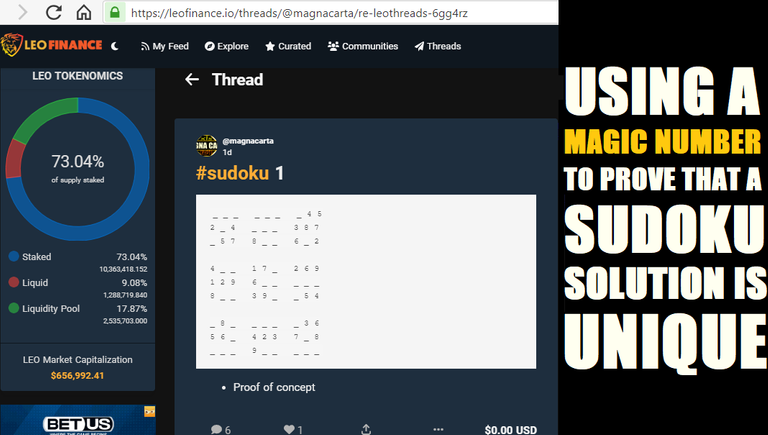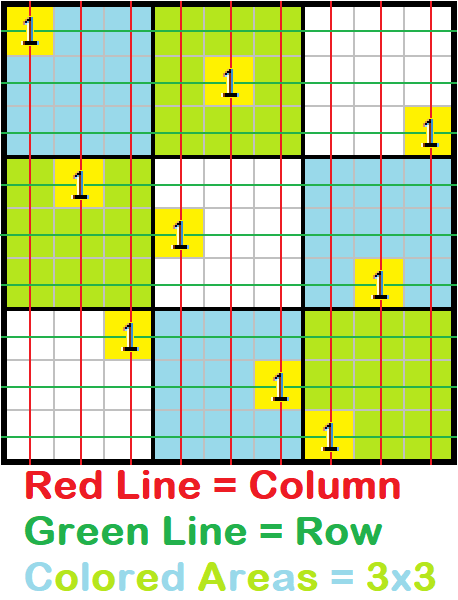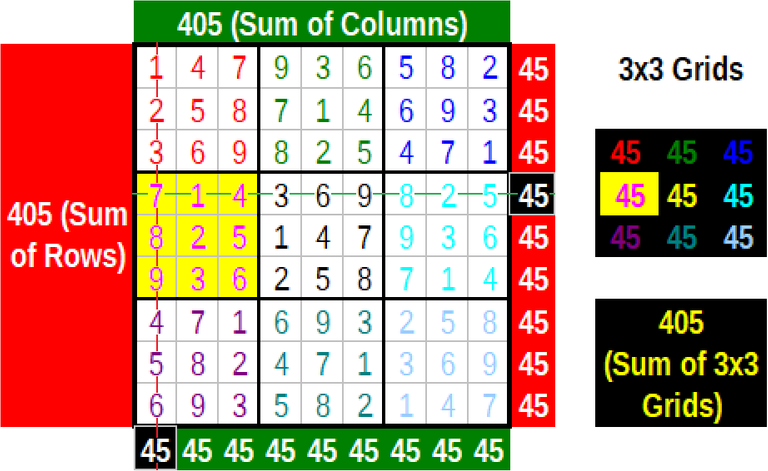# Using a Magic Number To Prove that a Sudoku Solution is Unique

in OCD3 months ago# Using a Magic Number To Prove that a Sudoku Solution is Unique

There are ways to confirm that a Sudoku puzzle solution is unique. One way features a Magic Number & pattern recognition.

Cover image and edits were made using MS Paint. Screen capture in cover image came from LeoThreads.

Figure images were made using Excel 2003, with edits made using MS Paint.

## Sudoku: A Logic Puzzle Using Numbers

Most of us are familar with crossword puzzles: Use provided clues to find a word which fits a group of squares.

Sudoku does something similar, only with numbers: Use given numbers as clues to guess which missing numbers go inside which open squares.

Even though Sudoku is a puzzle game using numbers, the puzzle is solved using logic rather than math. Replace each number with a letter or a color or a shape (or even team player position), and it is solved in the same manner: using logic.

Not only is the Sudoku puzzle solved using logic, but confirming that the solution is correct can also be done using logic. After a while, conscious use of logic leads to unconscious use of pattern matching. That leads to both faster solving and faster confirmation of solution.Figure 1: All digits must be unique back and forth along each row, up and down each column, and inside each 3x3 grid.

## Sudoku Puzzle Layout

Before trying to solve a Sudoku puzzle, it's important to understand how the puzzle is arranged:

• A 9x9 puzzle contains 81 numbers, some of which are given;
• The puzzle is organized in three ways:
• 9 rows;
• 9 columns; and
• 9 grids, each of which has 3 rows and 3 columns

Figure 1 above shows a mostly-empty Sudoku puzzle with a number used to show how a puzzle is laid out.

## Sudoku Rules

For anyone not familar with Sudoku, there isn't that much to learn or to know.

These are the only rules needed for solving a Sudoku puzzle:

• A number can only appear 1 time in each row.
• In each column, a number can only appear 1 time.
• No number can be repeated in a 3x3 grid

That's all that's expected from anyone solving a Sudoku puzzle.

## What about Sudoku Solving Strategies?

Many exist, including a few basic strategies. This post is about the completed Sudoku puzzle and how to determine if it is solved.

## No Math Calculations in Sudoku-- But Math Facts Exist Anyway

When the Sudoku rules noted above are followed, certain math facts reveal themselves. These math facts are the same no matter the standard puzzle solved and no matter who solves it.

Let's compare the rule with the math fact revealed by following that rule.

Rule 1 : A number can only appear 1 time in each row.
Math Fact 1 : The sum of all digits in a row is 45.

Below are equations which are TRUE, but only the final equation matters.

`````` 1 = 1
3 = 1 + 2
6 = 1 + 2 + 3
10 = 1 + 2 + 3 + 4
15 = 1 + 2 + 3 + 4 + 5
21 = 1 + 2 + 3 + 4 + 5 + 6
28 = 1 + 2 + 3 + 4 + 5 + 6 + 7
36 = 1 + 2 + 3 + 4 + 5 + 6 + 7 + 8
--------------------------------------
45 = 1 + 2 + 3 + 4 + 5 + 6 + 7 + 8 + 9
======================================
``````

No matter how the digits are arranged within the row, the sum of all 9 digits will always be 45.

Rule 2 : In each column, a number can only appear 1 time.
Math Fact 2 : The sum of all digits in a column is 45.

Math Fact for Rule 2 is the same as for Rule 1, so it won't be repeated here. The Magic Number is 45. If the sum is not this Magic Number, go back to the puzzle and fix the mistake.

Rule 3 : No number can be repeated in a 3x3 grid
Math Fact 3 : The sum of all digits in a 3x3 grid is 45.

A quick visual scan of the 3x3 grid should reveal these math facts:

• 4 2-digit sums of 10 plus the lone 5:
``````45 = (1+9) + (2+8) + (3+7) + (4+6) + 5
45 = 10 + 10 + 10 + 10 = 5
45 = 20 + 20 + 5
45 = 40 + 5
45 = 45
``````
• 3 sums of 15
``````45 = (1+2+3+4+5) + (6+9) + (7+8)
45 = 15 + 15 + 15
45 = 30 + 15
45 = 45
``````
• Sums of 10, 15, and 20
``````45 = (1+2+3+4) + (7+8) + (5+6+9)
45 = 10 + 15 + 20
45 = 25 + 20
45 = 45
``````

Other math facts may reveal themselves, but the point is that all unique digits add up to the Magic Number of 45.

Figure 2 below is a graphic representation of the above rules and math facts:Figure 2: All digits must be unique back and forth along each row, up and down each column, and inside each 3x3 grid.

## More Math Facts and Another Magic Number

Figure 2 above also notes a second Magic Number: 405

Using the above math facts, the match facts below reveal themselves:

• Math Fact 4: 45 for each row added 9 times equals 405
• Math Fact 5: 45 for each column added 9 times equals 405
• Math Fact 6: 45 for each 3x3 grid added 9 times equals 405

The sum of each row and column and 3x3 grid is equal to 45; after adding these sums, they add up to 405 for each grouping.

Think of the 405 as an additional check to confirm that the completed puzzle was solved correctly and arrived at a unique solution. With or without this additional layer of verification, 45 is the Magic Number to keep in mind.

## A Tool for Using the Magic Numbers

While it is possible to confirm the uniqueness of a Sudoku puzzle using the Magic Number and math facts explained earlier, it is more convenient to use a computerized tool to handle that task. A spreadsheet would be the perfect tool for this task.

While spreadsheets exist which do the solving if fed puzzle data, some people prefer to solve the puzzle themselves but don't want to spend time confirming the uniqueness of the solution.

Figure 2 above shows how a spreadsheet can be set up to handle the necessary calculations based on the rules of Sudoku and the math facts explained above. It's easy to set up a quick-and-dirty spreadsheet which can handle the drudgery of solution confirmation. It can also help train the eye to detect patterns based on the sequence of the digits used in the puzzle.

## Just My Two Sats

Even though Sudoku uses numbers, it is a game which relies on logic to arrive at a unique solution. The logic is derived from 3 simple rules:

• All digits in a row are unique;
• All digits in a column are unique; and
• All digits in a 3x3 grid are unique.

When these rules are followed, the Magic Number 45 appears as the sum for each row, column, and 3x3 grid.

(As an additional layer of verification, the sum of those sums is the Magic Number 405. The 9 row sums add up to 405. The 9 column sums add up to 405. The 9 3x3 grid sums add up to 405.)

To make scanning the numbers easier for verification of the unique solution, there are various ways to arrive at the Magic Number 45:

• 4 sums of 10 plus the lone 5;
• 3 sums of 15; and
• Sums of 10, 15, and 20.

Using the logic established helps with pattern recognition when trying to solve the puzzle. With better pattern recognition comes faster puzzle solving.

There may still be times when solving strategies are needed (especially for the more difficult puzzles), but even then solving time should be reduced when strategies are combined with improved pattern recognition.Do you like posting short bursts of text? Do it 240-characters at a time at LeoThreads using current UI or alpha-stage UI being tested.

Sort:

The rewards earned on this comment will go directly to the people ( magnacarta ) sharing the post on LeoThreads,LikeTu,dBuzz.

For this kind of topics I'd like to suggest StemSocial community next time. OCD community is for topics that don't fit in any other community and should be used when you don't find a niche community for your post.

Here's a guide I put together to help you learn about how communities work and why you should use them -> Communities Explained - Newbie Guide. I also put together a list of communities, which is not complete, there are much more communities on Hive, but it will help you get started.

Once you posted your post in the right community, you can then cross post it to OCD community. Here's a guide about cross posting.

Please don't delete any post with the purpose of reposting it in another community as that can be considered abuse. Leave this post here, you'll get it right next time.

Thank you for the clarification regarding what can be posted at OCD. I'll keep it in mind going forward.

You're welcome.Thanks for your contribution to the STEMsocial community. Feel free to join us on discord to get to know the rest of us!

Please consider delegating to the @stemsocial account (85% of the curation rewards are returned).

You may also include @stemsocial as a beneficiary of the rewards of this post to get a stronger support.